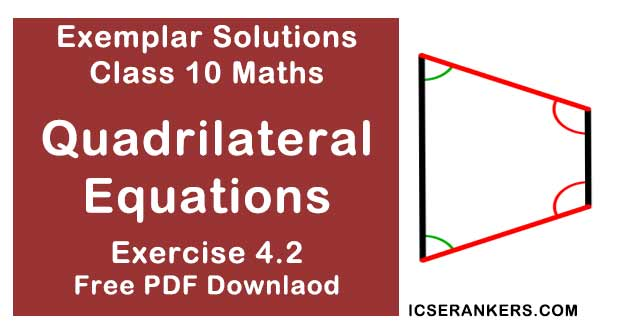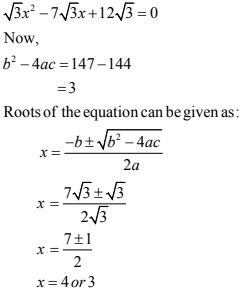# Chapter 4 Quadrilateral Equations NCERT Exemplar Solutions Exercise 4.2 Class 10 MathsChapter Name NCERT Maths Exemplar Solutions for Chapter 4 Quadrilateral Equations Exercise 4.2 Book Name NCERT Exemplar for Class 10 Maths Other Exercises Exercise 4.1Exercise 4.3Exercise 4.4 Related Study NCERT Solutions for Class 10 Maths

### Exercise 4.2 Solutions

(i) -3x + 4 = 0
(ii) 2x2 + x - 1 = 0
(iii) 2x2 - 6x + 9/2 = 0
(iv) 3x2 - 4x + 1 = 0
(v) (x + 4)2 - 8x = 0
(vi) (x - √2)2 - 2(x + 1) = 0
(vii) √2 x2 - (3/√2)x + 1/√2 = 0
(viii) x(1 -x) - 2 = 0
(ix) (x - 1)(x + 2) + 2 = 0
(x) (x + 1)(x - 2) + x = 0

Solution

(i) Equation x2 - 3x + 4 = 0 has no real roots.
D = b2 - 4ac
⇒ D = (-3)2 - 4(1)(4)
⇒ D = 9 - 16 < 0
So, the roots are imaginary.

(ii) Equation 2x2 + x - 1 = 0 has two real and distinct roots.
D = b2 - 4ac
⇒ D = 12 - 4(2)(-1)
⇒ D = 1 + 8> 0
So, the roots are real and distinct.

(iii) Equation 2x2 - 6x + (9/2) = 0 has real and equal roots.
D = b2 - 4ac
⇒ D = (-6)2 - 4(2)(9/2)
⇒ D = 36 - 36 = 0
So, the roots are real and equal.

(iv) Equation 3x2 - 4x + 1 = 0 has two real and distinct roots.
D = b2 - 4ac
⇒ D = (-4)2 - 4(3)(1)
⇒ D = 16 - 12 > 0
So, the roots are real and distinct.

(v) Equation (x + 4)2 - 8x = 0 has no real roots.
Simplifying the above equation,
x2 + 8x + 16 - 8x = 0
⇒ x2 + 16  = 0
D = b2 - 4ac
⇒ D = (0) - 4(1)(16) < 0
So, the roots are imaginary.

(vi) Equation (x - √2)2 - √2(x + 1) = 0  has two distinct and real roots.
Simplifying the above equation,
x2 - 2√2x + 2 - √2x - √2 = 0
⇒ x2 - √2(2 + 1)x + (2 - √2) = 0
⇒ x2 - 3√2x + (2 - √2) = 0
D = b2 - 4ac
⇒ D = (-3√2)2 - 4(1)(2 - √2)
⇒ D = 18 - 8 + 4√2 > 0
So, the roots are real and distinct.

(vii)  Equation √2x2  - 3x/√2 + 1/2 = 0 has two real and distinct roots.
D = b2 - 4ac
⇒ D = (-3/√2 )2 - 4(√2)(1/2)
⇒ D = (9/2) - 2√22 > 0
So, the roots are real and distinct.

(viii) The equation x(1 - x) - 2 = 0 has no real roots.
Solving the above equation,
x2 - x + 2 = 0
D = b2 - 4ac
⇒ D = (-1)2 - 4(1)(2)
⇒ D = 1 - 8 < 0
So, the roots are imaginary,

(ix) Equation (x - 1)(x + 2) + 2 = 0 has two real and distinct roots.
Solving the above equation,
x2 + x = 0
D = b2 - 4ac
⇒ D = 12 - 4(1)(0)
⇒ D = 1 - 0 > 0
So, the roots are real and distinct.

(x) Equation (x + 1)(x - 2) + x = 0 has two real and distinct roots.
Solving the above equation,
x2 + x - 2x -2 + x = 0
⇒ x2 - 2 = 0
D = b2 - 4ac
⇒ D = (0)2 - 4(1)(-2)
⇒ D = 0 + 8 > 0
So, the roots are real and distinct.

2. Write whether the following statements are true or false. Justify your answers.
i. Every quadratic equation has exactly one root.
ii. Every quadratic equation has at least one real root.
iii. Every quadratic equation has at least two roots.
iv. Every quadratic equations has at most two roots.
v. If the coefficient of x2 and the constant term of a quadratic equation have opposite signs, then the quadratic equation has real roots.
vi. If the coefficient of x2  and the constant term have the same sign and if the coefficient of x term is zero, then the quadratic equation has no real roots.

Solution
(i) False.
For example, a quadratic equation x2 - 16 = 0 has two distinct roots – 4 and 4.

(ii) False.
For example, equation x2 + 6 = 0 has nor real root.

(iii) False.
For example, a quadratic equation x2 - 4x + 4 = 0 has only one root which is 2.

(iv) True,
Because every quadratic polynomial has almost two roots.

(v) True,
Because in this case discriminant is always positive.
For example, in ax2 + bx + c = 0, as a and c have opposite sign, ac <  0
Discriminant = b2 - 4ac > 0.

(vi) True,
Because in this case discriminant is always negative.
For example, in ax2 + bx + c = 0, as b = 0, and a and c have same sign then ac > 0
Discriminant = b2 - 4ac = -4ac < 0

Solution

No, a quadratic equation with integral coefficients may or may not have integral roots.
Explanation:
Consider the following equation,
16x2 - 4x - 2 = 0
The roots of the given equation are 1/2  and -1/4 which are not integers.
Therefore, a  quadratic equation with integral coefficient can or cannot have integral roots.

4. Does there exist a quadratic equation, whose coefficients are rational both of its roots are irrational? Justify your answer.

Solution

Yes, a quadratic equation having coefficients as rational number, has irrational roots. Taking example,
2x2 - 3x - 15 = 0 has rational coefficients.
Now, calculating,So, we can say that roots are irrational as √129 is irrational.

5. Does there exist a quadratic equation whose coefficient are all distinct irrationals but both the roots are rational ? why ?

Solution

Yes, there may be a quadratic equation whose coefficient are all distinct irrationals, but both the roots are rational.
For example, consider a quadratic equation having distinct irrational coefficients.
In this equation, the roots are real.Hence, the roots are rational while coefficients a, b, c were irrational.

6. Is 0.2 a root of equation x2 - 0.4 = 0 ? Justify your answer.

Solution
If we consider 0.2 as a root of equation x2 - 0.4 = 0, then 0.2 must satisfy the given equation.
x2 - 0.4 = 0
⇒ (0.2)2 - 0.4 = 0
⇒ 0.04 - 0.4 = 0
⇒ -0.36 ≠ 0
Therefore, 0.2 is not a root of the given equation.

7. If b = 0, c < 0, is it true that the roots of x2 + bx + c = 0 are numerically equal and opposite in sign ? Justify you answer.

Solution
Equation is x2 + bx + c = 0
b = 0   [Given]
So, x2 + c = 0
x2 = -c
⇒ x = √-c
Since, c is negative so -c becomes positive or √-c is real.
So, the roots of the given equation are
x = +√-c
and
x =√-c  [where (-c) is positive]
Therefore, the roots of the given equation are real, equal and opposite in sign.